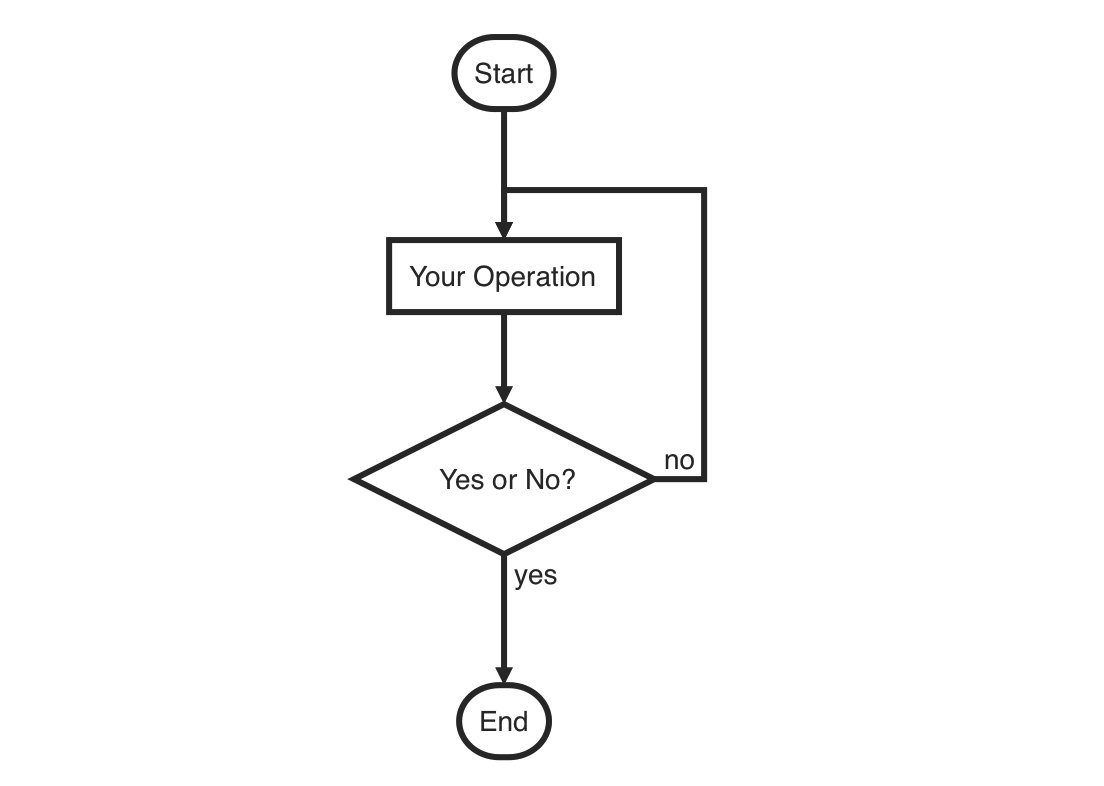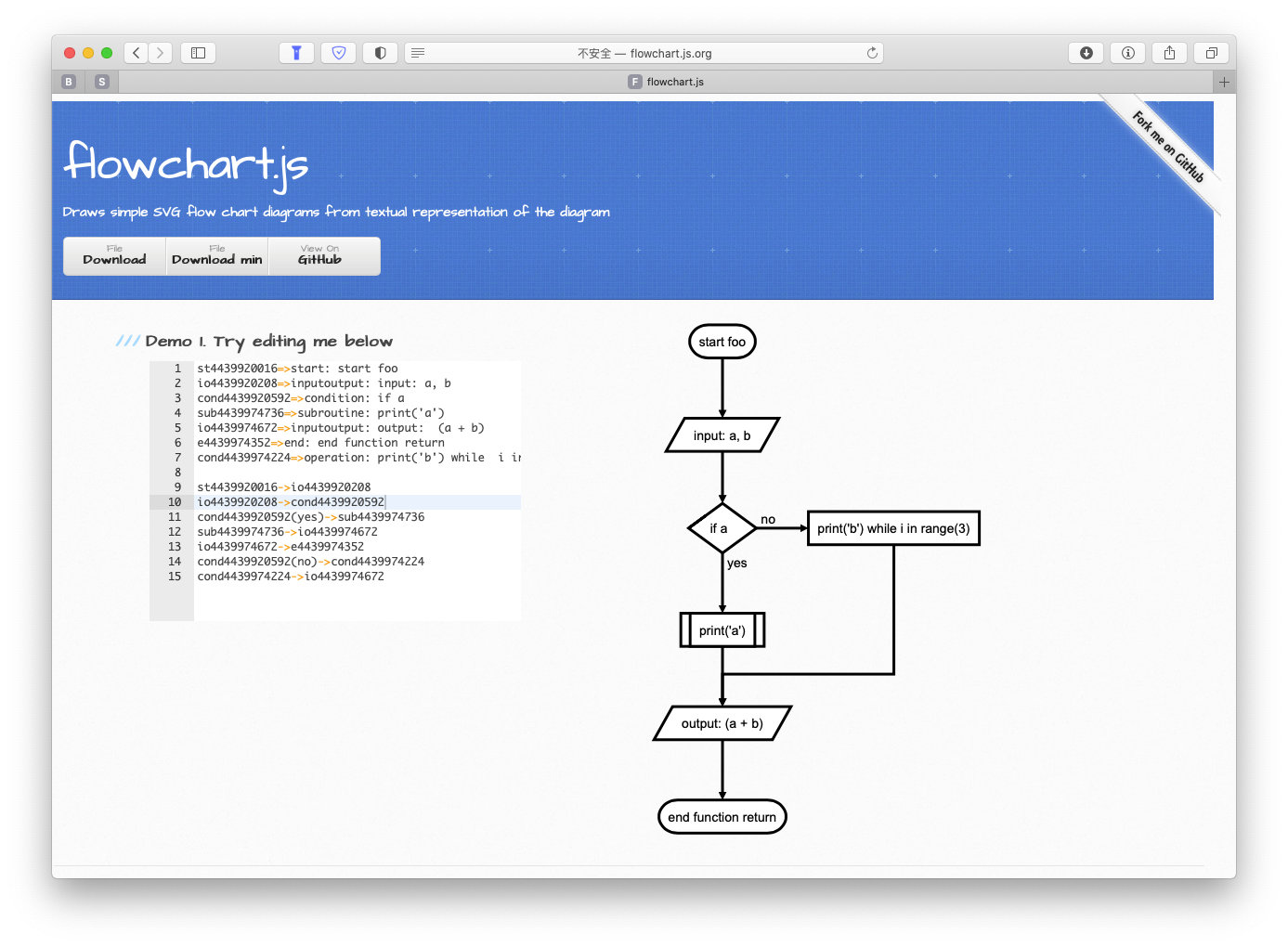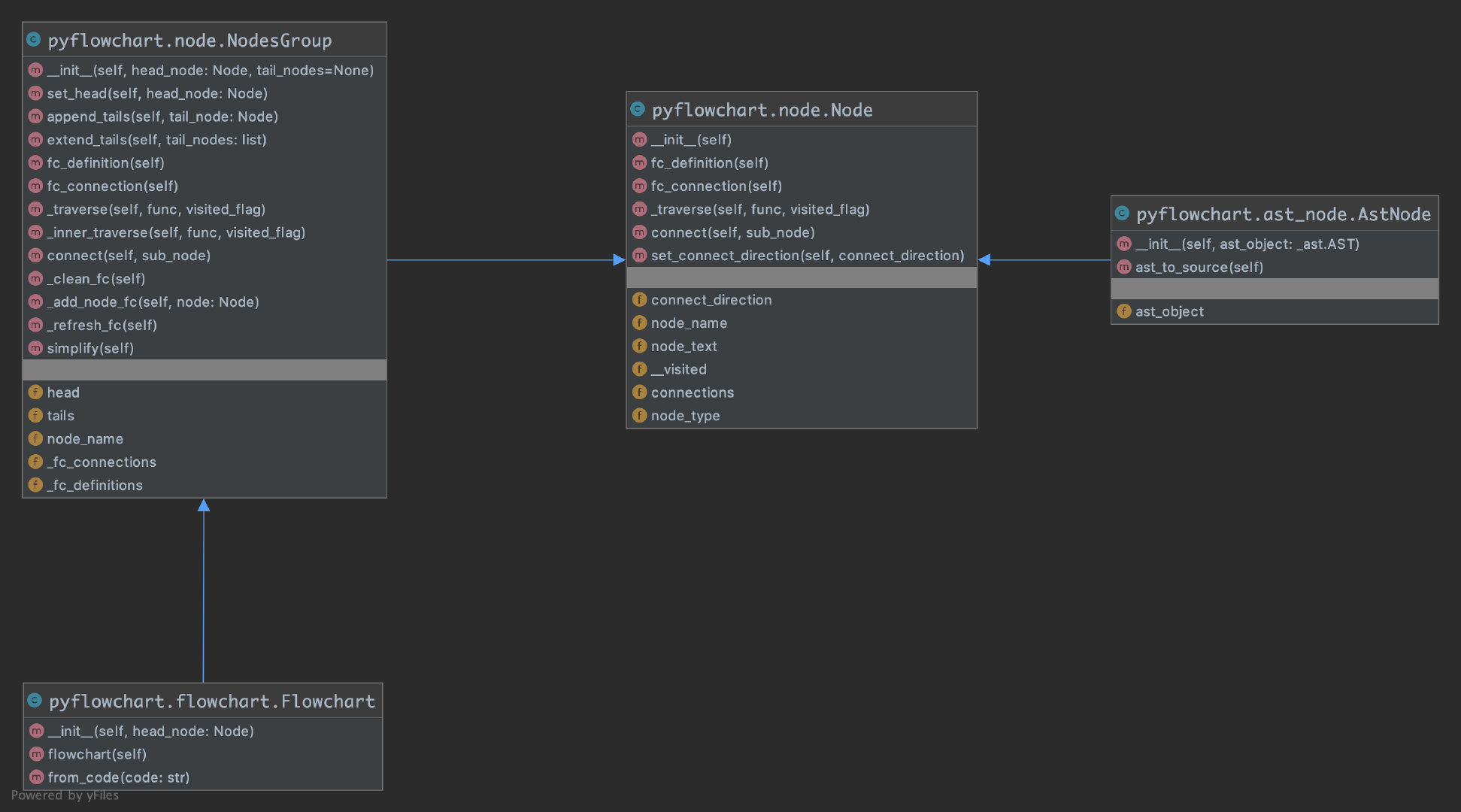• 时间:

# Python 代码转流程图

（本来我是取名叫做 PyFlow 的，上传 PyPi 的时候发现重名了🤦‍♂️。所以改成了 PyFlowchart）

## flowchart.js

``````st=>start: Start
op=>operation: Your Operation
cond=>condition: Yes or No?
e=>end

st->op->cond
cond(yes)->e
cond(no)->op
``````## PyFlowchart

``````\$ pip3 install pyflowchart
``````

``````def foo(a, b):
if a:
print("a")
else:
for i in range(3):
print("b")
return a + b
``````

``````\$ python3 -m pyflowchart simple.py
``````

``````st4439920016=>start: start foo
io4439920208=>inputoutput: input: a, b
cond4439920592=>condition: if a
sub4439974736=>subroutine: print('a')
io4439974672=>inputoutput: output:  (a + b)
e4439974352=>end: end function return
cond4439974224=>operation: print('b') while  i in range(3)

st4439920016->io4439920208
io4439920208->cond4439920592
cond4439920592(yes)->sub4439974736
sub4439974736->io4439974672
io4439974672->e4439974352
cond4439920592(no)->cond4439974224
cond4439974224->io4439974672
``````## 实现原理

PyFlowchart 利用 Python 内置的 ast 包，把代码转化成 AST（抽象语法树），然后把 AST 转化成自己定义的 Node 组成的图，每个 Node 对应一个 flowchart 中的 node，遍历这个图就可以得到流程图了。

``````>>> import ast
>>> expr = """
... def add(a, b):
...     return a + b
... """
>>> expr_ast = ast.parse(expr)
>>> expr_ast
<_ast.Module object at 0x10c773e10>
>>> ast.dump(expr_ast)
# p.s. 这里手动做了格式化
Module(body=[FunctionDef(name='add',
args=arguments(
args=[
arg(arg='a', annotation=None),
arg(arg='b', annotation=None)],
vararg=None, kwonlyargs=[], kw_defaults=[], kwarg=None, defaults=[]),
body=[Return(value=BinOp(
left=Name(id='a', ctx=Load()),
op=Add(),
right=Name(id='b', ctx=Load())))],
decorator_list=[],
returns=None)])
```````Node` 是最最基础的类，表示流程图中的一个节点，其他一切都继承自它。Node 有节点类型、名称、内容等属性，提供的 `fc_definition()``fc_connection()` 方法可以把自己转化为 flowchart 语言的字符串。另外的 `__visited``_traverse()` 是用来遍历图的。与 flowchart 中的 node types 对应，我们实现了各种 Node 的子类：StartNode、EndNode、OperationNode…

`NodesGroup` 是一个特殊的 Node，它自己不会出现在生成的 flowchart 中，但它可以包含一些其他 Node。这个设计是受到 Android 的 View、ViewGroup 的启发，有了这个 NodesGroup，if 语句、for / while 循环这样有嵌套的 body 的情况就很容易处理了。

`AstNode` 表示一个从 AST 对象得到的 Node。构造 AstNode，就是把某个 AST 对象翻译成一个 Node（也可以是 NodesGroup）。其子类就和各种 ast 对象对应（也就和 Python 的各种语句对应）： If、Loop、Return …

`Flowchart` 代表一张流程图。流程图就是一堆连在一起的节点嘛，所以 Flowchart 是 NodesGroup 的子类。在其 `from_code()` 中方法中，实现了用 ast 包解析 Python 代码，得到 ast 对象的工作。在 `flowchart()` 方法中，遍历图，拿到所有节点的 flowchart 表示，汇总成一张完整的 flowchart 流程图。

• Flowchart 中利用 ast 包实现了 code to ast；
• AstNode 及其子类实现了 ast to node graph；
• Node 及其子类实现了 node graph to flowchart。

• https://github.com/cdfmlr/pyflowchart/``````by('CDFMLR', '2020.10.24')
# 🎉
``````